Function Repository Resource:

# NthSubset

Get the subset of a list for a given index

Contributed by: Wolfram Staff (original content by Sriram V. Pemmaraju and Steven S. Skiena)
 ResourceFunction["NthSubset"][n,list] gives the nth subset of list in canonical order.

## Examples

### Basic Examples (1)

The first 16 subsets of a list:

 In:=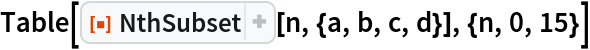Out=### Properties and Relations (3)

NthSubset does not sort its input, so the ordering of subsets is based on positions in the original list:

 In:=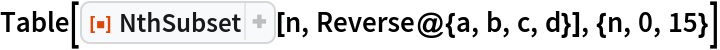Out=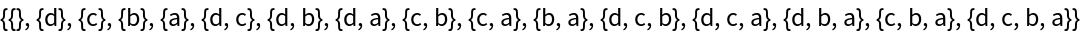The 69,381st subset returned by Subsets:

 In:=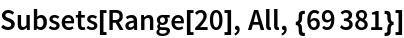Out=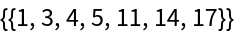Or by the zero-indexed NthSubset:

 In:=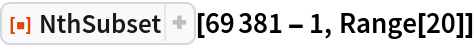Out=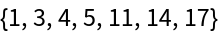The ordering of subsets is based on length (smaller subsets come first), followed by lexicographic list positions. This is not the fastest ordering for computing subsets:

 In:=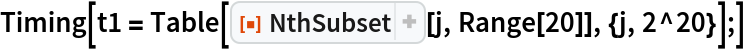Out=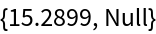A faster enumeration can be attained if one imposes only lexicographic position ordering:

 In:=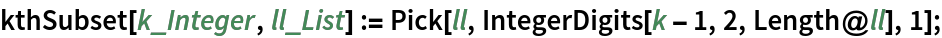In:=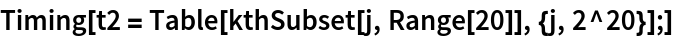Out=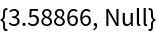Check that these have the same subsets:

 In:=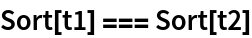Out=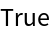## Version History

• 1.0.0 – 04 March 2020